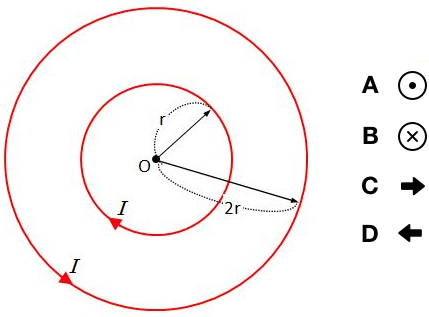# Magnetic field of loop currentThe above shows two loop currents with radii of $r$ and $2r,$ respectively, on the same plane. The current intensities are the same, which is $I,$ and flow in opposite directions. What is the correct direction of the magnetic field at position $O$ which is the center of the two loops?

Note: A($\odot$) means magnetic field into the plane, and B($\otimes$) means magnetic field out from the plane.

×